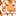# New to Qlik Sense

If you’re new to Qlik Sense, start with this Discussion Board and get up-to-speed quickly.

Announcements
QlikWorld 2023, a live, in-person thrill ride. Save \$300 before February 6: REGISTER NOW!
cancel
Showing results for
Did you mean:Contributor

## Comparaison des Mois et Mois Year-1

Bonjour,

Je me permets de vous écrire suite à une difficulté que j’ai rencontré lors de la comparaison des  valeurs de Nbr logements sur la période YearTodate(Max(month)) par rapport à Nbr logements sur la même période (même nombre de mois ) sur  Year -1.

Labels (2)

• ### Visualization

1 Solution

Accepted SolutionsChampion III

YearToDate - Current Year

=sum({<Datefield={">=\$(=Date(yearstart(Max(Datefield))))<=\$(=Date(Max(Datefield)))"}>}SomeMeasure)

YearToDate - Previous Year

MonthToDate - Current Year

=sum({<Datefield={">=\$(=Date(monthstart(Max(Datefield))))<=\$(=Date(Max(Datefield)))"}>}SomeMeasure)

MonthToDate - Previous Year

Vineeth Pujari
If a post helps to resolve your issue, please accept it as a Solution.
2 RepliesChampion III

YearToDate - Current Year

=sum({<Datefield={">=\$(=Date(yearstart(Max(Datefield))))<=\$(=Date(Max(Datefield)))"}>}SomeMeasure)

YearToDate - Previous Year

MonthToDate - Current Year

=sum({<Datefield={">=\$(=Date(monthstart(Max(Datefield))))<=\$(=Date(Max(Datefield)))"}>}SomeMeasure)

MonthToDate - Previous Year